Definitions of Square Dance Calls and Concepts

Index -->  Plus  |  A1  |  A2  |  C1  |  C2  |  C3A  |  C3B  |  C4  |  NOL  |
Definitions (Text Only) -->  Plus  |  A1  |  A2  |  C1  |  C2  |  C3A  |  C3B  |  C4  |  NOL  |
 Find call:

 view (admin)

\$BJ?9T\$J(B Wave, Eight Chain Thru, \$B\$^\$?\$O(B Thar \$B\$+\$i(B.

Arm Turn 1/2 \$B\$r\$7\$F(B; Centers \$B\$O(B Trade and Spread \$B\$r\$7(B, Ends \$B\$O(B U-Turn Back \$B\$r\$7\$F(B Crossover Circulate \$B\$r\$7\$^\$9(B.

\$BJ?9T\$J(B Wave \$B\$^\$?\$O(B Eight Chain Thru \$B\$+\$i\$O(B, \$BJ?9T\$J(B Two-Faced Line \$B\$G=*\$o\$j\$^\$9(B.

Parallel Waves \$B\$+\$i(B: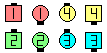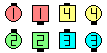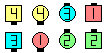Swing-O-Late\$B\$NA0(B Arm Turn 1/2\$B\$N8e(B Centers Trade & Spread as Ends U-Turn Back &Crossover Circulate\$B\$N8e(B (\$B=*\$o\$j(B)
Thar \$B\$+\$i(B: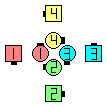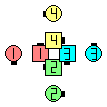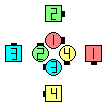Swing-O-Late\$B\$NA0(B Arm Turn 1/2\$B\$N8e(B Centers Trade & Spread as Ends U-Turn Back &Crossover Circulate\$B\$N8e(B (\$B=*\$o\$j(B)

\$BCmSwing-O-Late \$B\$O(B \$B1&

anything O-Late [obsolete]: anything \$B\$N%3!<%k\$r\$7\$F(B; Centers \$B\$O(B Spread \$B\$r\$7(B, Ends \$B\$O(B U-Turn Back \$B\$r\$7\$F(B Crossover Circulate \$B\$r\$7\$^\$9(B.Choreography for Swing-O-LateComments? Questions? Suggestions?

https://www.ceder.net/def/swingolate.php?level=master&language=japan
22-June-2021 16:48:28
Copyright © 2021 Vic Ceder.  All Rights Reserved.